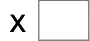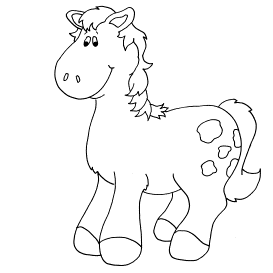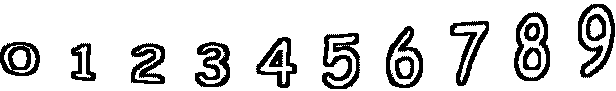3= 6   10= 402= 20   3= 15   2= 4   9= 63 2= 12   9= 9   8= 8   8= 72